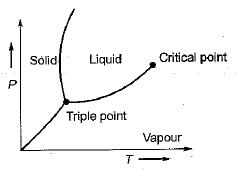# Properties Of Pure Substance - 1

## 10 Questions MCQ Test Topicwise Question Bank for GATE Mechanical Engineering | Properties Of Pure Substance - 1

Description
This mock test of Properties Of Pure Substance - 1 for Mechanical Engineering helps you for every Mechanical Engineering entrance exam. This contains 10 Multiple Choice Questions for Mechanical Engineering Properties Of Pure Substance - 1 (mcq) to study with solutions a complete question bank. The solved questions answers in this Properties Of Pure Substance - 1 quiz give you a good mix of easy questions and tough questions. Mechanical Engineering students definitely take this Properties Of Pure Substance - 1 exercise for a better result in the exam. You can find other Properties Of Pure Substance - 1 extra questions, long questions & short questions for Mechanical Engineering on EduRev as well by searching above.
QUESTION: 1

Solution:
QUESTION: 2

Solution:
QUESTION: 3

### The slope of sublimation curve on P-Tdiagram for all substances is

Solution:QUESTION: 4

In P-V-Tsurface, the zone below the triple point is known as

Solution:
QUESTION: 5

For which of the following substances, the gas laws can be used with minimum error?

Solution:
QUESTION: 6

The point that connects the saturated liquid line to the saturated vapour line is called

Solution:
QUESTION: 7

At triple point of a pure substance

Solution:

The Triple point is a line on the P - v diagram where all the three phases solid, liquid and gases exist in equilibrium. At a pressure below the triple point line, the substance cannot exist in liquid phase and the substance when heated, transforms from solid to vapour by absorbing the latent heat of sublimation from the surroundings.

QUESTION: 8

During throttling process

Solution:
QUESTION: 9

Sublimation curve on P-T diagram for all substances possesses the following slope

Solution:

This is true for all substances.

QUESTION: 10

At critical point the enthalpy of vapourization is

Solution:

At critical point enthalpy of vapourization is zero i.e. liquid can directly converted into vapour (without any latent heat transaction).